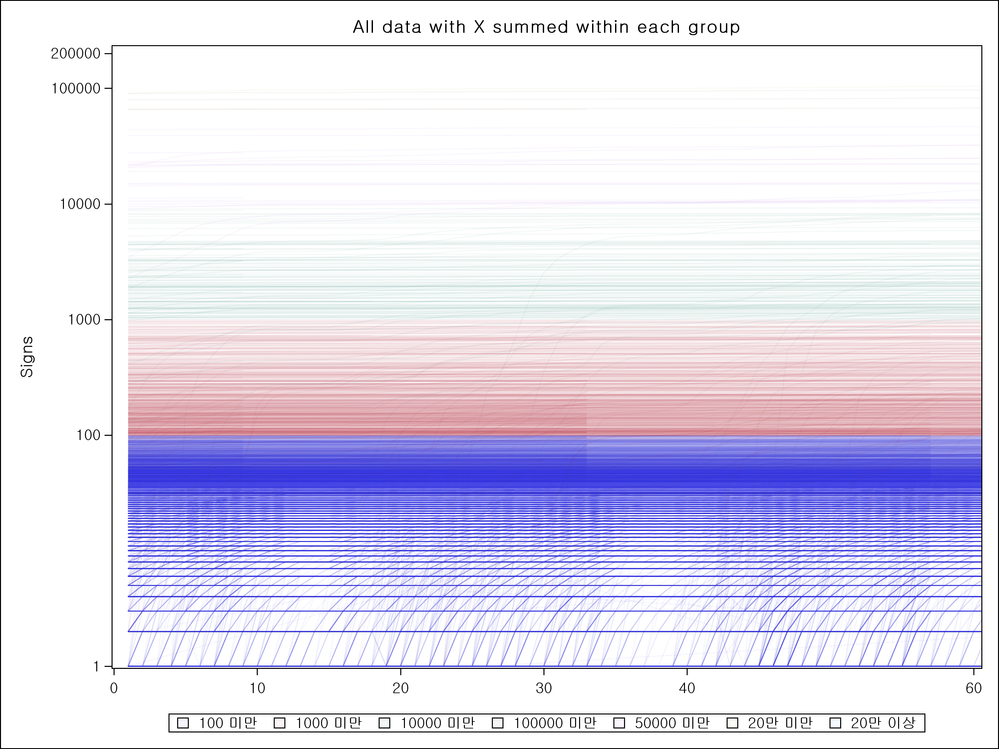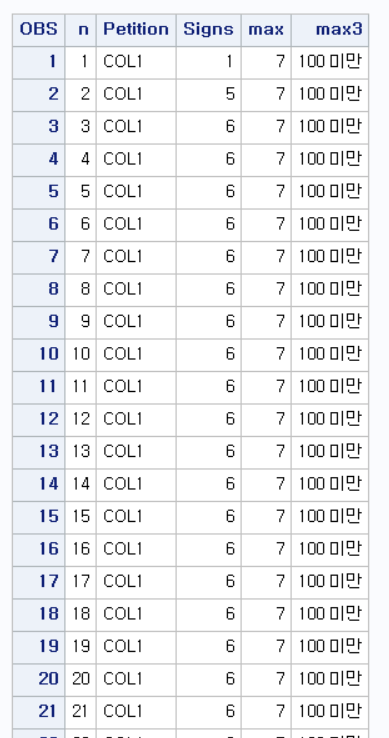## Is there a way to set different transparency per groups i sgplot?

Hi.

I'm working on how to set different transparency per groups. I searched in google and SAS help, and tried to use legenditem and discreteattrmap (which was showing red characters... I don't know why. I just copied that from SAS Help book examples).

Anyway, I got nothing.

Here's the code for my sgplot. (Thanks to Rick's advice and tips for spaghetti plot)

``````	proc sgplot data=pilot.pilot9;
label Signs="Signs" Petition="Petition";
series x=n y=Signs / group=Petition grouplc=max3 lineattrs=(pattern=solid) transparency=0.95 ;
xaxis display = (nolabel) values=(10 20 30 40 0 60);
yaxis label="Signs" type=log logbase=10 logstyle=logexpand values=(1 100 1000 10000 100000 200000) gridattrs=(pattern=dash color=black);
keylegend / type=linecolor title="";
run;``````

And here's the result of the graph.As you can see, most of graphs are included under 100 or 1000, so I tried to set those group with 0.98 of transparency while others have 0.5 or 0.2 of transparencies. So that I can catch which one just popped up from the low signs to higher.

(FYI, I am using SAS 9.4)

Thank you.

9 REPLIES 9

## Re: Is there a way to set different transparency per groups i sgplot?

You could use multiple SERIES statements in the same PROC SGPLOT, with different transparency levels. In order for this to work, you have to rearrange your input data set so that the X and Y variables in each group are present with unique names, while they are missing for the other groups. So, for example, using SASHELP.CLASS, the input data set to PROC SGPLOT would look like this (where I am using SCATTER, but it works with SERIES):

``````data have;
set sashelp.class sashelp.class(rename=(height=height1 weight=weight1));
/* Jitter the data in height1 and weight1 so they can be different, otherwise we won't see them on the plot */
/* This is needed for this example and not for your real data */
weight1=weight1+5*rand('normal');
height1=height1+5*rand('normal');
run;

proc sgplot data=have;
scatter x=height y=weight/transparency=0.2;
scatter x=height1 y=weight1/transparency=0.8;
run;``````
--
Paige Miller

## Re: Is there a way to set different transparency per groups i sgplot?

Thank you, and sorry for the late reply.

Rearranging the data is hard for me but it seems I have no choice. I'll give it a try!

Thank you!DanH_sas
SAS Super FREQ

## Re: Is there a way to set different transparency per groups i sgplot?

For the record, FILLTRANSPARENCY and MARKERTRANSPARENCY are supported in discrete attribute maps, which would give you individual transparency per group value (but not for LINEs currently). However, if you CAN use attribute maps to control the color per group in your case (if you don't care about specific colors per group, use the STYLESATTRS statement instead). If you adjust the lightness/saturation in the colors per group, the single transparency value might still work for you.

Hope this helps!

Dan

## Re: Is there a way to set different transparency per groups i sgplot?

Thank you DanH.

Looks like I have to take your way.

However, I merely begin with attribute map, it will be a long shot.

But adjusting lightness/saturation is quite a new way for me. I think it would be the solution (if I can deal with it).

Wish me luck! I'll search for the post about attrmap and lightness/saturation adjustment.

## Re: Is there a way to set different transparency per groups i sgplot?

@MonoLight wrote:

Thank you, and sorry for the late reply.

Rearranging the data is hard for me but it seems I have no choice. I'll give it a try!

Why is it hard? I gave an example of rearranging an existing data set to accomplish this purpose.

--
Paige Miller

## Re: Is there a way to set different transparency per groups i sgplot?The data has about 70 "n"s.

And COL1~COL33600.

And it's sorted like the screenshot above. So there are about 70 * 33600 rows in there.

I'm figuring out how to make this one into your setting.

## Re: Is there a way to set different transparency per groups i sgplot?

If the values of PETITION go from COL1 to COL33600, you'd probably need a macro to create the data as needed to graph them with separate transparencies.

The macro would loop through all of the COL1 through COL33600, modifying the data set as I described in my original method. You'd also have to have 33600 SERIES statements, each with its own transparency level. Depending on the speed and memory of your computer, this could take quite a while to run.

--
Paige Miller

## Re: Is there a way to set different transparency per groups i sgplot?

Oh I forgot to mention.

I got COL1 ~33600 for Petition, but I also made the variable(max3) which group them in 7 categories.

So I hope I can use that to draw a graph setting 7 different transparency. But I'm not sure I can do it.

Actually I got headache in whole weekend (maybe because of SAS) so I'll try it tomorrow with all advice.

Making macro is beyond my skills, so maybe other way would be needed.

But thanks for considering my problem, really!

Also if you got some other advice popped up, please let me know!

## Re: Is there a way to set different transparency per groups i sgplot?

For seven categories, I would not even think about a macro, just brute force program this as in my earlier example. So

``````data have2;
set have(where=(max3=1) rename=(n=n1 signs=signs1))
have(where=(max3=2) rename=(n=n2 signs=signs2))
have(where=(max3=3) rename=(n=n3 signs=signs3))
have(where=(max3=4) rename=(n=n4 signs=signs4))
have(where=(max3=5) rename=(n=n5 signs=signs5))
have(where=(max3=6) rename=(n=n6 signs=signs6))
have(where=(max3=7) rename=(n=n7 signs=signs7));
run;

proc sgplot data=have2;
scatter x=n1 y=signs1/transparency=0.2;
scatter x=n2 y=signs2/transparency=0.8;
/* Additional scatter statements go here, I left them out to save me some typing */
run;``````

where I assume the values of max3 are 1 to 7 (if they are not 1 to 7, then make the obvious change to the code above).

--
Paige Miller
Discussion stats
• 9 replies
• 2284 views
• 3 likes
• 3 in conversation